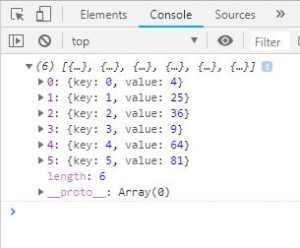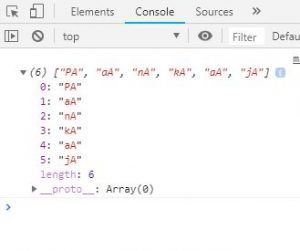# JavaScript | Array map() Method

• Difficulty Level : Medium
• Last Updated : 21 Jul, 2021

The map() method in JavaScript creates an array by calling a specific function on each element present in the parent array. It is a non-mutating method. Generally map() method is used to iterate over an array and calling function on every element of array.
Syntax:

`array.map(function(currentValue, index, arr), thisValue)`

Parameters: This method accepts two parameters as mentioned above and described below:

Hey geek! The constant emerging technologies in the world of web development always keeps the excitement for this subject through the roof. But before you tackle the big projects, we suggest you start by learning the basics. Kickstart your web development journey by learning JS concepts with our JavaScript Course. Now at it's lowest price ever!

• function(currentValue, index, arr): It is required parameter and it runs on each element of array. It contains three parameters which are listed below:
• currentValue: It is required parameter and it holds the value of current element.
• index: It is optional parameter and it holds the index of current element.
• arr: It is optional parameter and it holds the array.
• thisValue: It is optional parameter and used to hold the value of passed to the function.

Return Value: It returns a new array and elements of arrays are result of callback function.

Below examples illustrate the use of array map() method in JavaScript:
Example 1: This example use array map() method and return the square of array element.

 ```<``html``>    ``<``head``>``    ``<``title``>``        ``JavaScript Array map() Method``    `````` ` `<``body``>``    ``<``div` `id``=``"root"``>``     ` `    ````    ``<``script``>``     ` `        ``var el = document.getElementById('root');``        ``var arr = [2, 5, 6, 3, 8, 9];``         ` `        ``var newArr = arr.map(function(val, index){``            ``return {key:index, value:val*val};``        ``})``         ` `        ``console.log(newArr)``         ` `        ``el.innerHTML = JSON.stringify(newArr);``    `````` ` `            `

Output:Example 2: This example use array map() method to concatenate character ‘A’ with every character of name.

 ```<``html``>``     ` `<``head``>``    ``<``title``>``        ``JavaScript Array map() Method``    `````` ` `<``body``>``    ``<``div` `id``=``"root"``>`` ` `    ``<``script``>``     ` `        ``var el = document.getElementById('root');``        ``var name = "Pankaj";``         ` `        ``// New array of character and names ``        ``// concatenated with 'A' ``        ``var newName = Array.prototype.map.call(name, function(item) {``            ``return item + 'A';``        ``})``         ` `        ``console.log(newName)``    `````` ` `                    `

Output:Example 3: This example use array map() method to return the square of array elements.

 ```<``html``>``<``head``>``    ``<``title``>``        ``JavaScript Array map() Method``    `````` ` `<``body``>``    ``<``div` `id``=``"root"``>`` ` `    ``<``script``>``        ``var el = document.getElementById('root');``         ` `        ``// Map is being called directly on an array``        ``// Arrow function is used``        ``el.innerHTML = [6, 7, 4, 5].map((val)=>{ ``            ``return val*val;``        ``})``    `````` ` ``

Output:

`36, 49, 16, 25`

JavaScript is best known for web page development but it is also used in a variety of non-browser environments. You can learn JavaScript from the ground up by following this JavaScript Tutorial and JavaScript Examples.

My Personal Notes arrow_drop_up